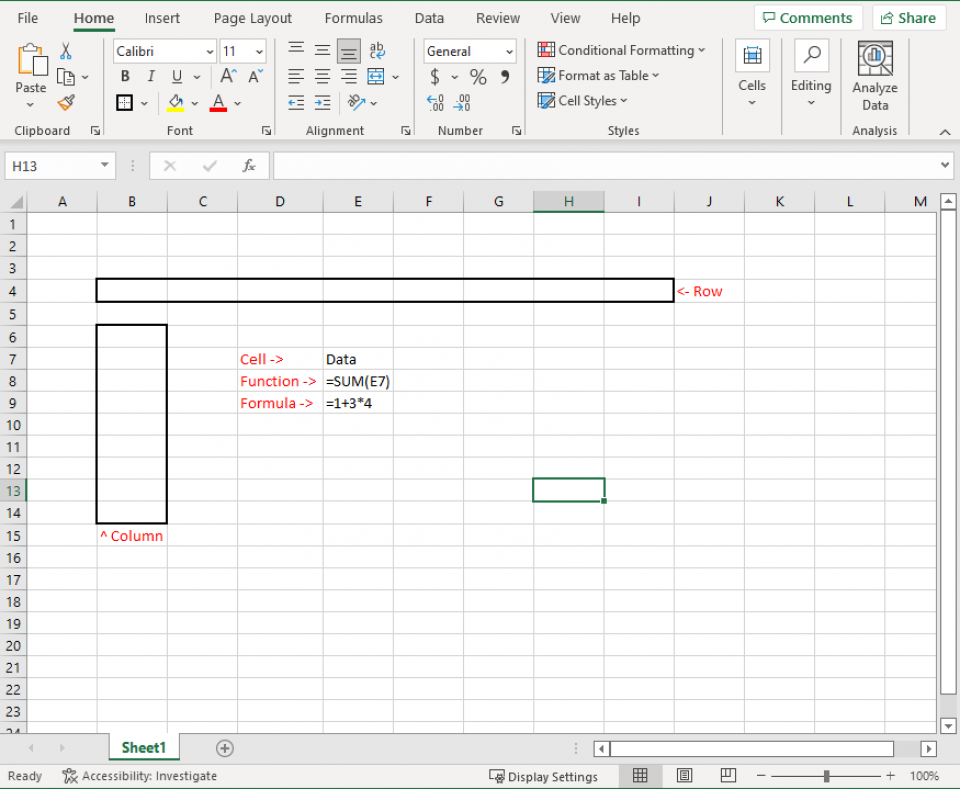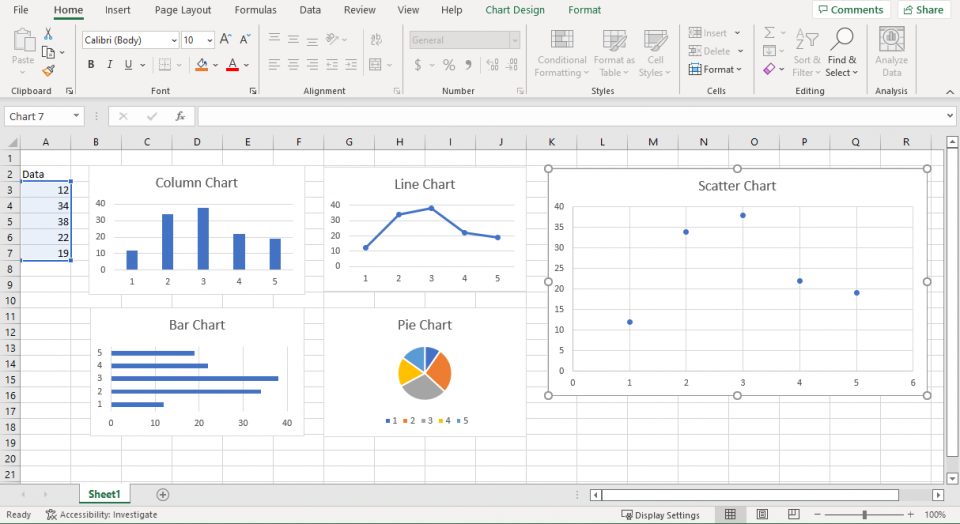Need a discount on popular programming courses? Find them here. View offers

Don't have an account?

Have you read our submission guidelines?

Go back to

Microsoft Excel is one of the top in-demand skills on the market. Whether you're starting to learn Excel or already an expert, having a cheat sheet in your pocket can help. An Excel cheat sheet gives you a brief overview of the functions, commands, formulas, and shortcuts in Excel—so you can focus on your work.

Let’s start with some of the basic terminology used in Excel so you can thrive in countless industries, including data analytics and finance. We will also cover the anatomy of a spreadsheet, relevant courses, and important functions. Need to buy a copy? You can get Excel from Microsoft.

### Basic Terminology

Review your basic vocabulary before looking at functions and commands to fully understand how Excel formats its data.

 Active Cell The currently active cell in the worksheet Cell An individual box in the worksheet that can contain data, text, or a formula Column A vertical group of cells in the worksheet. Columns are identified by letters (A, B, C, etc.) Formula A set of instructions that performs calculations on values in the worksheet Function A predefined formula is used to perform standard calculations, such as summing a range of values Row A horizontal group of cells in the worksheet. Rows are identified by numbers (1, 2, 3, etc.) Worksheet Also known as a spreadsheet; the grid of columns and rows that you can enter in Excel

### The Anatomy of an Excel Sheet### Excel Data Types

As a spreadsheet program, Excel focuses on storing data. Here are the data types that Excel frequently stores.

 Text Also called labels, text values identify data in a worksheet or store things like names and descriptions. Example: Hello, World! Numbers Numbers are used for calculations and can be formatted as currency, percentages, decimals, etc. Example: 1.12 Dates/Times Dates and times track and calculate data over time. Example: 2022-02-02 Logical Values Logical values are either true or false. Example: TRUE, FALSE Arrays Array formulas perform calculations on a range of cells simultaneously. Example: SUM(A1:A4)

### Common Excel Functions

In Excel, a function is simply a preset formula or algorithm. They help parse information and display it for a variety of uses. Here are some of the most common Excel formulas in our Excel functions list cheat sheet.

 SUM Adds the values of a range of cells Example: SUM(A1:A4) SUMIFS Sums values that meet specific criteria. Example: SUMIFS(A1:A4,B1:B4,E1) AVERAGE Calculates the average values in a range of cells Example: AVERAGE(A1:A4) COUNT Counts the number of cells in a range that contains numbers Example: COUNT(A1:A4) MIN Finds the smallest value in a range of cells Example: MIN(A1:A4) MAX Finds the largest value in a range of cells Example: MAX(A1:A4) TRIM Removes all white space from the front and back of a cell. Example: TRIM(A1) IF Checks whether a condition is met and returns one value if true and another if false Example: IF(A1=‘Yes’, True, False) CONCATENATE Combines the values of multiple cells into a single cell. Example: CONCATENATE(A1, B1) VALUE Convert numbers that have been stored in text to integers. Example: VALUE(B1) MIN Finds the minimum value of a set. Example: MIN(A1:O1) MAX Finds the maximum value of a set. Example: MAX(A1:O1) PROPER Formats text with the correct capitalization; useful when importing data from other sources. Example: PROPER(A1) CEILING Round a number up to the first number of significance, e.g. 39.1 to either 39 or 40. Example: CEILING(A1,4) FLOOR Round a number down to the first number of significance, e.g. 39.12 to either 39.1 or 39. Example: FLOOR(A1,4) LEN Return the number of characters in a string, useful for data validation. Example: LEN(A1) NOW Get the current date and time. Note that it will return the time of the system you’re on. Example: NOW() TODAY Similar to now, but this just gives the date, rather than the date and time. You can also use DAY(), MONTH(), and YEAR(). Example: TODAY()

### Advanced Excel Functions Cheat Sheet

These advanced Excel functions can be a little more difficult to use—but they’re sophisticated methods of processing and analyzing data.

 VLOOKUP Looks up a value in the leftmost column of a table and returns a corresponding value from another column Example: VLOOKUP(“Text”,A1:C4,2,FALSE) INDEX Performs a lookup based on a row and column number instead of a lookup value Example: INDEX(A1:A4,1,1) HLOOKUP Looks up a value in the top row of a table and returns a corresponding value from another row in the table Example: HLOOKUP(“Text”, A1:C4, 2)

### Excel Shortcuts

Shortcuts are a great way to increase productivity. Once shortcuts become a habit, you’ll find even simpler tasks faster. Here are some of the most common keyboard shortcuts to help you work faster in Excel.

 F2 Edit the active cell. F5 Go to a specific cell in the worksheet. CTRL + Arrow Move to the edge of the worksheet’s data. Shift + F11 Insert a new sheet. Alt + = Sum the cells. Ctrl + Shift + “+” Insert a new row/column. Ctrl + “-” Delete a row/column. Ctrl + “*” Select all cells with formulas. Ctrl + ‘ Copy the value from above a cell. Alt + Enter Insert a line break in a cell.

### Excel Commands

In addition to shortcuts, Excel has many built-in commands that can be accessed using shortcut keys or the ribbon. Here are some of the most common.

 Paste Special Opens the Paste Special dialog box, which allows you to choose how to paste data from the clipboard Format Painter Copies formatting from one cell and applies it to another cell or range of cells Fill Handle Allows you to quickly fill a range of cells with data that follows a pattern AutoSum Automatically calculates the sum of a range of selected cells Sparklines Creates small, graphical representations of data in a single cell

### Excel Graphs

Not everything is readable in a series of columns and rows. When you need something human-readable, you need a graph. Excel offers several ways to create graphs and charts. Here are some of the most common.

 Column Charts Used to compare data points side-by-side. Bar Charts Used to compare data points side-by-side. Line Charts Used to show trends over time. Pie Charts Used to show percentages or proportions. Scatter Plots Used to show relationships between data points.### Tips and Tricks

Finally, here are a few tips and tricks to help you work faster in Excel.

1. Learn how to use keyboard shortcuts. Keyboard shortcuts can save you a lot of time working in Excel. Pressing CTRL+C will copy the selected cells, while CTRL+V will paste them.
2. Use the AutoFill feature when filling in similar values. The AutoFill feature in Excel is handy for filling in a data series. If you have a list of months, you can use AutoFill to fill in the days of the month automatically.
3. Use conditional formatting to increase the readability of your sheet. Conditional formatting allows you to highlight cells that meet specific criteria. You could use conditional formatting to highlight all cells that contain a value greater than 10.
4. Use data validation to ensure your sheet includes the right data types. You could use data validation to ensure cells only contain numbers—or only dates.
5. Memorize the most common and useful formulas. Formulas are one of the most powerful features in Excel, but they can take some time to learn.
6. Consider using macros. Macros are small programs that you can create to automate tasks in Excel. You could create a macro that automatically inserts the current date when you open a workbook or a macro that automatically saves and closes your files.
7. Get comfortable with pivot tables. Pivot tables are a great way to summarize large amounts of data and sort it.
8. Don’t be afraid of filters. Filters let you view only the data that you want to see. For example, you could use a filter only to view the data for a specific month.

## Conclusion

A basic Excel cheat sheet or Excel commands cheat sheet can only do so much. It offers a quick alternative to building everything from scratch, and it helps users get started quickly. However, all spreadsheet users should learn the basics of Excel. Once you understand the capabilities, you should be able to look up things you need to know in the above Excel cheat sheet.

#### 1. How Do You Use the Cheat Sheet in Excel?

Use the above Excel function cheat sheet whenever you need to do something specific in Excel. You can review Excel cheat sheet formulas to refresh your memory or use CTRL+F to find a specific area of the Excel formula cheat sheet.

#### 2. What are the 5 Functions in Excel?

Here’s a quick list of five basic Excel functions:

• VLookup Formula
• Text to Columns
• Duplicate Removal
• Pivot Tables
• Concatenate Formula

#### 3. What is the Fastest Way to Learn Excel Formulas?

Most formulas that you would need will pop up when you start typing in Excel. As long as you know what formulas are available in Excel, you should be able to look them up on this sheet.

#### 4. What are the 7 Basic Excel Formulas?

The seven basic Excel formulas are SUM, AVERAGE, MIN, MAX, COUNT, COUNTA, and VLOOKUP. These are just a few of the many formulas available in Excel.

5. Where do you get Excel?

You can buy a copy of Excel from Microsoft as a stand-alone piece of software or get it as part of the Microsoft 365 suite of products. While Google offers a free alternative with their powerful sheets service, the original software has additional features professionals use every day.

STAY IN LOOP TO BE AT THE TOP

#### Welcome to the club and Thank you for subscribing!

By Jenna Inouye

Jenna Inouye has been a full stack developer for two decades, specializing in web application design and development. For the last eight years, she has worked as a news and feature writer focusing on technology and finance, with bylines in Udemy, SVG, and The Gamer.

View all post by the author

Disclosure: Hackr.io is supported by its audience. When you purchase through links on our site, we may earn an affiliate commission.